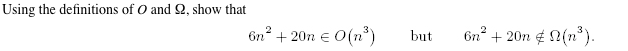# Question & Answer: Using the definitions of O and Ohm show that 6n^2 + 20 n elementof (n^3) but 6n^2 + 20 NotElement n Ohm (n^3)…..Using the definitions of O and Ohm show that 6n^2 + 20 n elementof (n^3) but 6n^2 + 20 NotElement n Ohm (n^3).

Don't use plagiarized sources. Get Your Custom Essay on
Question & Answer: Using the definitions of O and Ohm show that 6n^2 + 20 n elementof (n^3) but 6n^2 + 20 NotElement n Ohm (n^3)…..
GET AN ESSAY WRITTEN FOR YOU FROM AS LOW AS \$13/PAGE

Definition of O and  :

• O : The function f(n) = O(g(n)) iff there exists positive constants C and n0 such that f(n) <= C*g(n) for all n > n0.

Here , f(n)= 6n2 + 20n

6n2 + 20 n <= 10* n3 n > 1

Here , g(n) = n3

C= 10, n0 = 1, both are positive constants.

f(n)  O(n3)

•  : A function f(n) = (g(n)) iff there exists positive constants C and n0 such that f(n) >=C* g(n)  n>=n0

Here f(n) = 6n2 + 20n

6n2 + 20n >= n3 when n > 1 && n<6. But for values of n>6, this doesn’t hold true.

Here g(n) = n3

C=1

f(n) (n3) .

Whereas, 6n2 + 20n > 6n2 ,  n>1

Here g(n) = n2

C = 6 and n0 = 1.

f(n) (n2)

Therefore, 6n2 + 20n = O(n3) but 6n2 + 20n  (n3) .